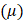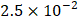# The ratio of electron and hole current in a semiconductor is 7/4 and the ratio of drift velocities of electrons and holes is 5/4, then ratio of concentrations of electrons and holes will be a) 5/7 b) 7/5 c) 25/49 d) 49/75

## Question ID - 151441 :- The ratio of electron and hole current in a semiconductor is 7/4 and the ratio of drift velocities of electrons and holes is 5/4, then ratio of concentrations of electrons and holes will be a) 5/7 b) 7/5 c) 25/49 d) 49/75

3537

 (b)orIf the amplification factor of a triodeis 22 and its plate resistance is, then the mutual conductance of this valve is mho is a)b)c)d)